# Class 6 Maths NCERT Solutions Chapter 1 – Knowing our Numbers

Class 6 Maths NCERT Solutions Chapter 1 – Knowing our Numbers comprises of the 3 Exercises,

This Chapter contains the Exercises relating to the following topics , which are discussed in Chapter 1 – Number System Class 6 NCERT book  : –

• Exercise 1.1 Introduction
• Exercise 1.2 Comparing Numbers
• Exercise 1.2.1 How many numbers can you make?
• Exercise 1.2.2 Shifting digits
• Exercise 1.2.3 Introducing 10,000
• Exercise 1.2.4 Revisiting place value
• Exercise 1.2.5 Introducing 1,00,000
• Exercise 1.2.6 Larger numbers
• Exercise 1.2.7 An aid in reading and writing large numbers
• Exercise 1.3 Large Numbers in Practice
• Exercise 1.3.1 Estimation
• Exercise 1.3.2 Estimating to the nearest tens by rounding off
• Exercise 1.3.3 Estimating to the nearest hundreds by rounding off
• Exercise 1.3.4 Estimating to the nearest thousands by rounding off
• Exercise 1.3.5 Estimating outcomes of number situations
• Exercise 1.3.6 To estimate sum or difference
• Exercise 1.3.7 To estimate products
• Exercise 1.4 Using Brackets
• Exercise 1.4.1 Expanding brackets
• Exercise 1.5 Roman Numerals

Class 6 Maths NCERT Solutions Chapter 1 Exercise 1.1 – Video Explanation

Class 6 Maths NCERT Solutions Chapter 1 Exercise 1.2 – Video Explanation

Class 6 Maths NCERT Solutions Chapter 1 Exercise 1.3 – Video Explanation

### Class 6 Maths NCERT Solutions Chapter 1 – Exercise 1.1

This Page 1 of Exercise 1.1, contains the following questions and their solutions  : –

• Conversion of numbers from lakhs, millions, crores into ten thousand, hundred thousand , ten lakh etc ;
• Placing Commas and writing the numerals ;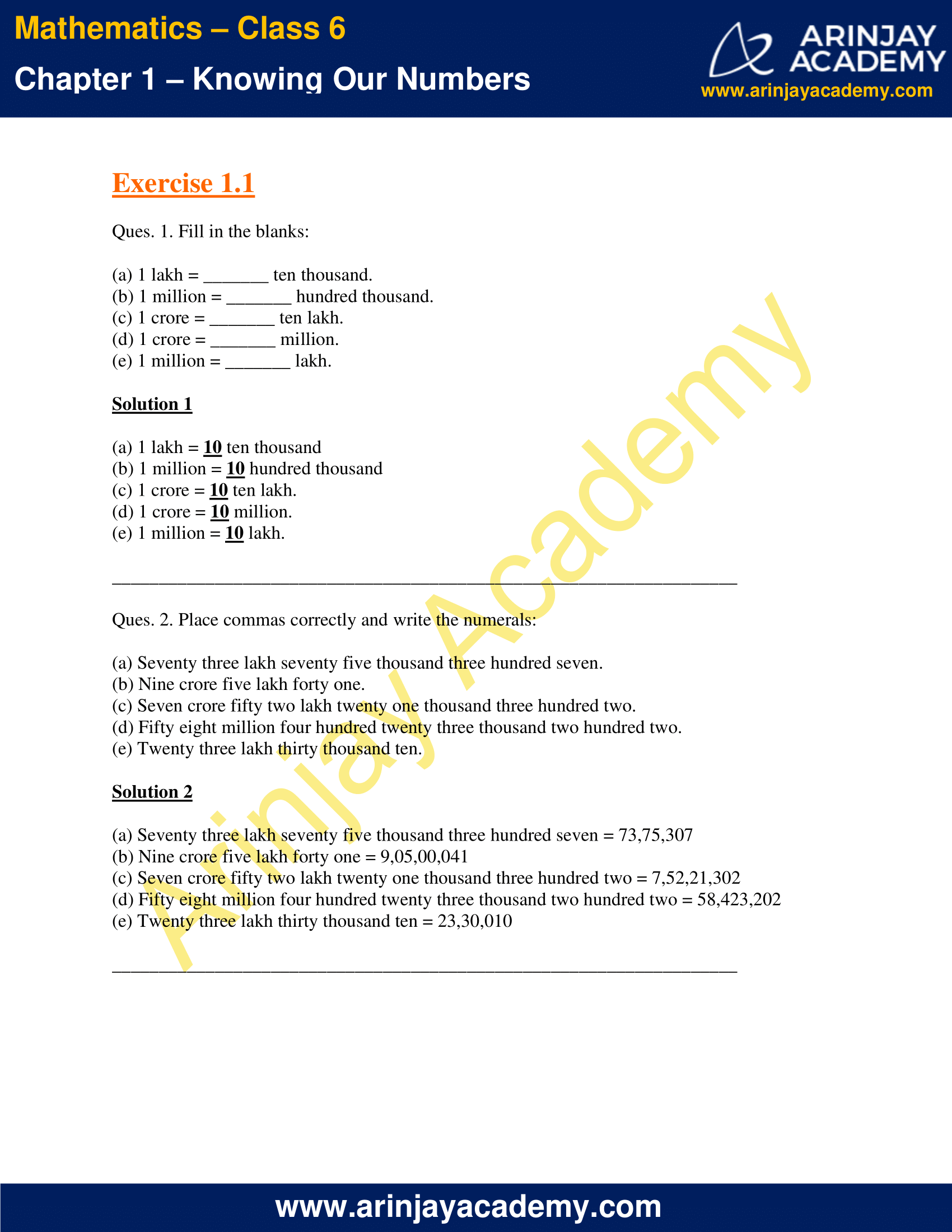This Page 2 of Exercise 1.1, contains the following questions and their solutions  : –

• Placing Commas and writing according to the Indian System of Numeration  ;
• Placing Commas and writing according to the International System of Numeration .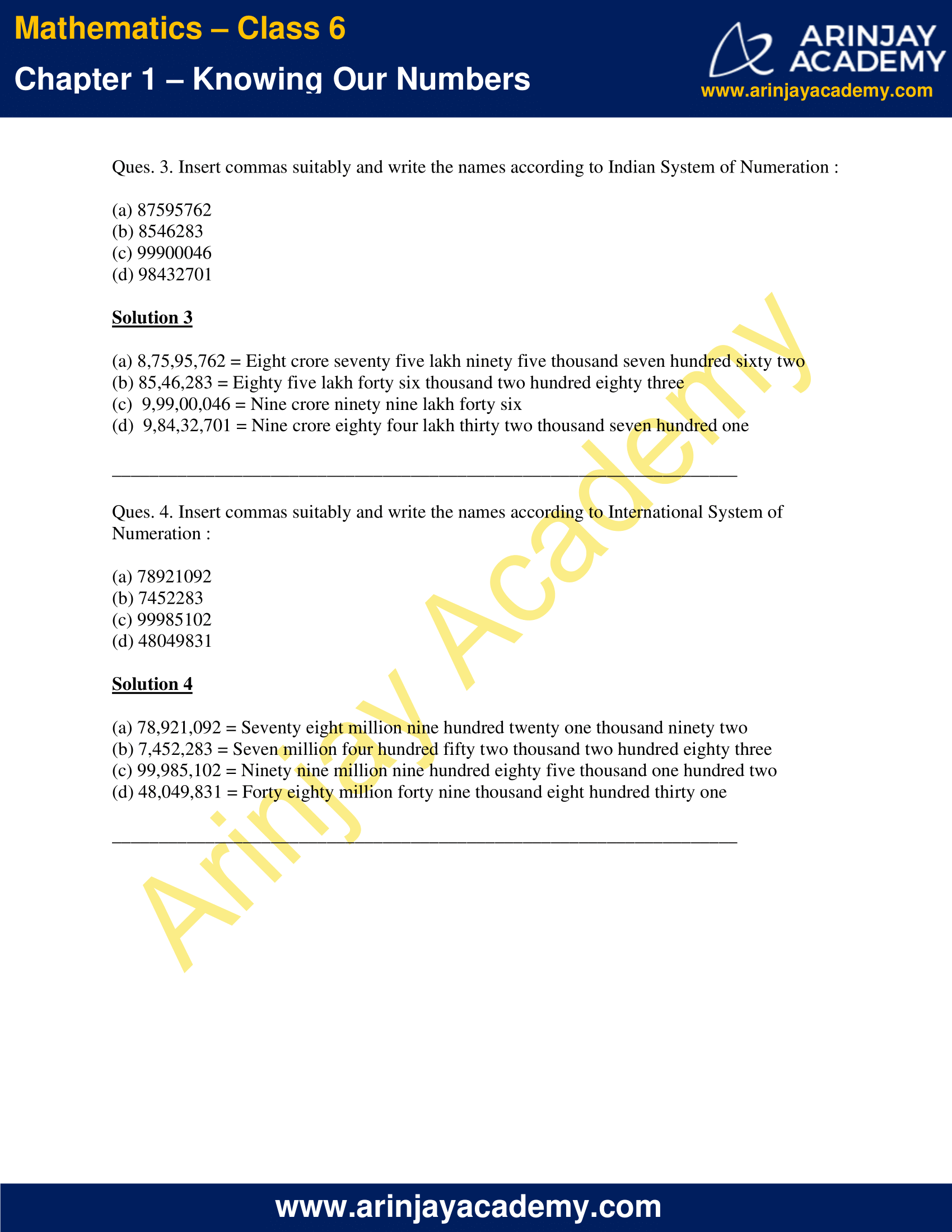This Page 3, contains the following questions and their solutions  : –

• Conversion of numbers from lakhs, millions, crores into ten thousand, hundred thousand, ten lakh, etc ;
• Placing Commas and writing the numerals ;

### Class 6 Maths NCERT Solutions Chapter 1 – Exercise 1.2

This Page 1 of Exercise 1.2, contains the following questions and their solutions  : –

• Finding the total number of tickets sold in an exhibition when the tickets sold on four separate days were given ;
• Runs to be scored by a cricketer to reach 10,000 runs ;
• The margin of victory of an election candidate when votes secured by both candidates are given.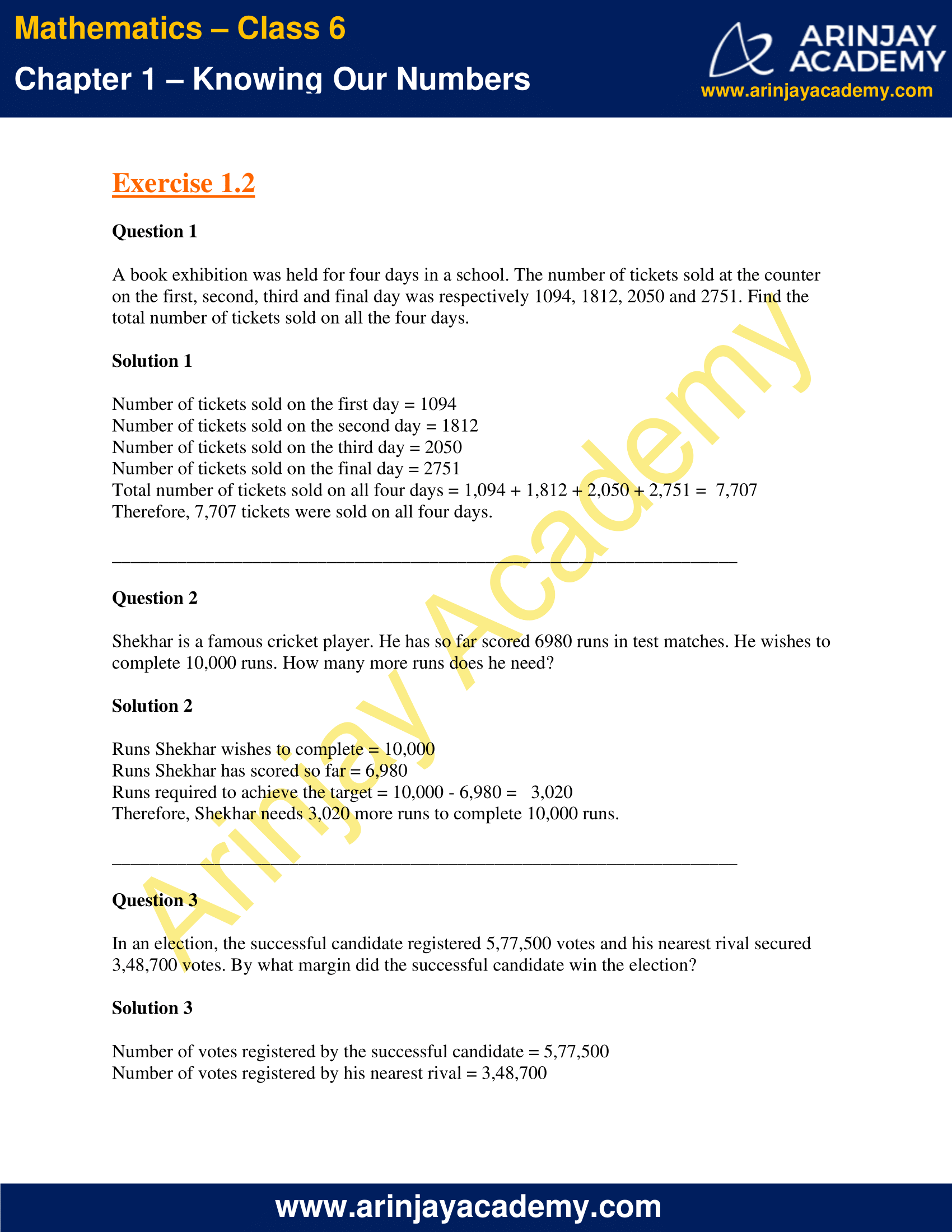This Page 2 of Exercise 1.2, contains the following questions and their solutions  : –

• Finding total number of books sold by a store ;
• Largest and smallest digits that can be written from a given set of numbers  and difference between them .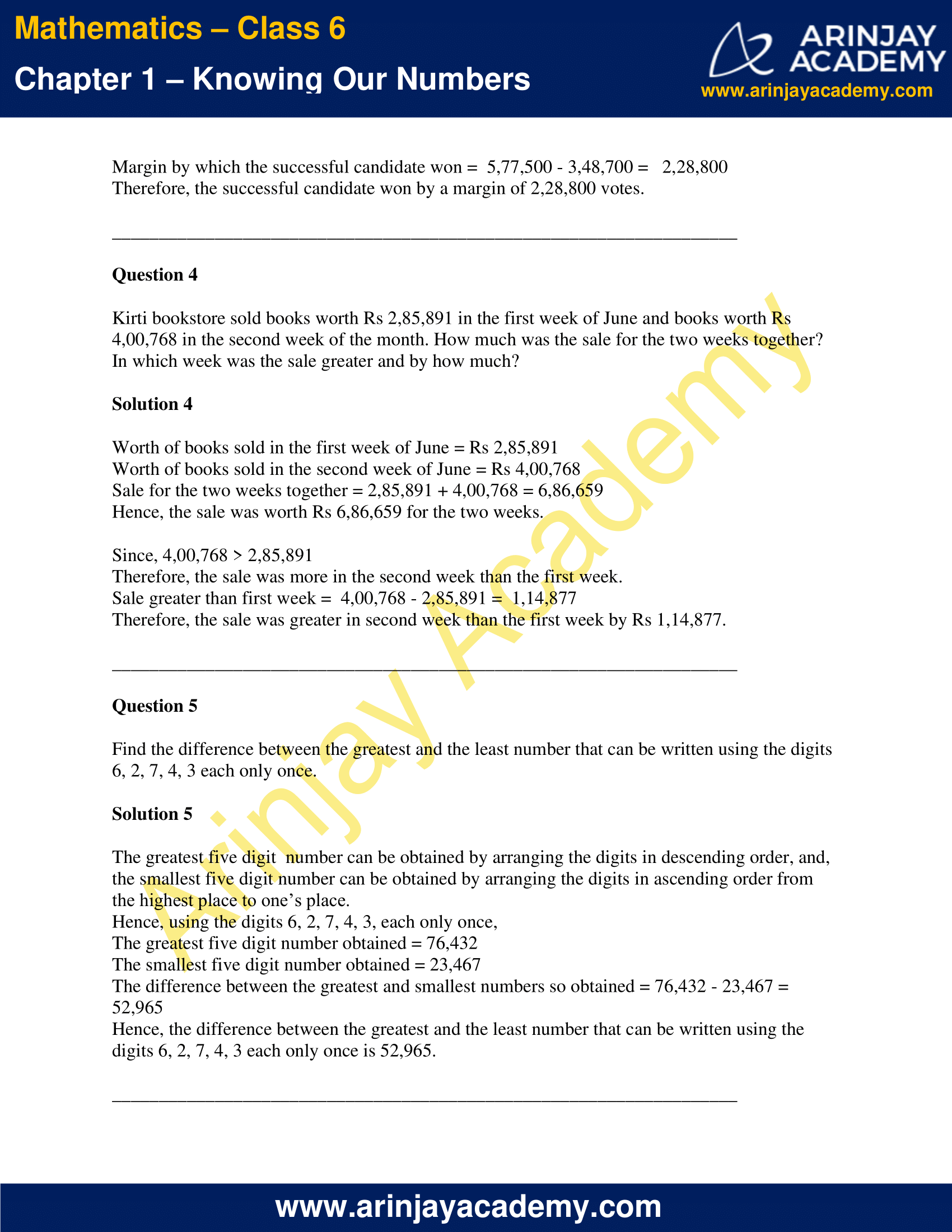This Page 3 of Exercise 1.2, contains the following questions and their solutions  : –

• Finding the number of screws manufactured by a machine in a month when the number of screws manufactured in a day are given
• Calculating money left with the merchant who spent part of the money with her on purchasing certain machinery
• Finding the difference between product computed by a student, if he multiplied a given number by 56 instead of 65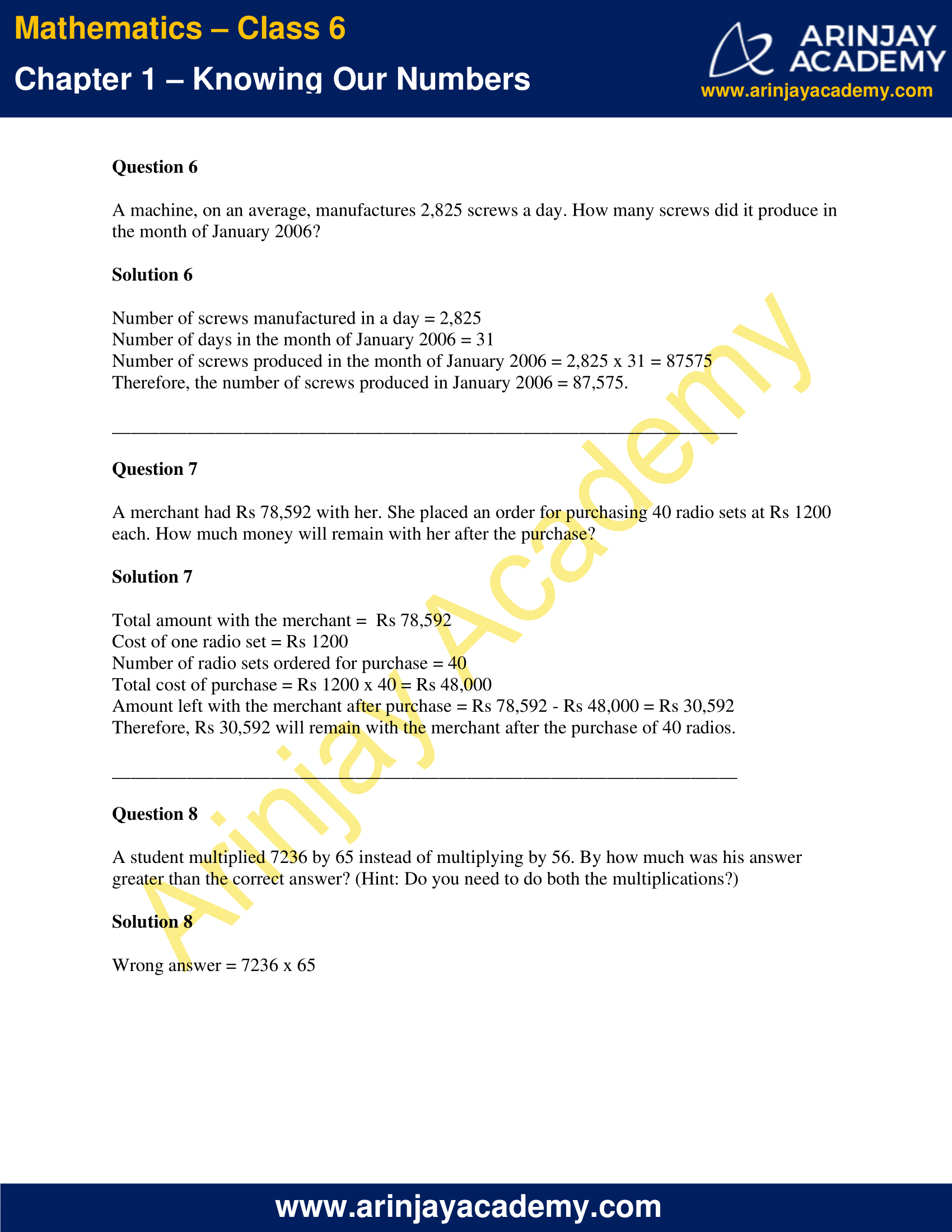This Page 4 of Exercise 1.2, contains the following questions and their solutions  : –

• Finding number of shirts which can be stitched from given amount of cloth, and remaining cloth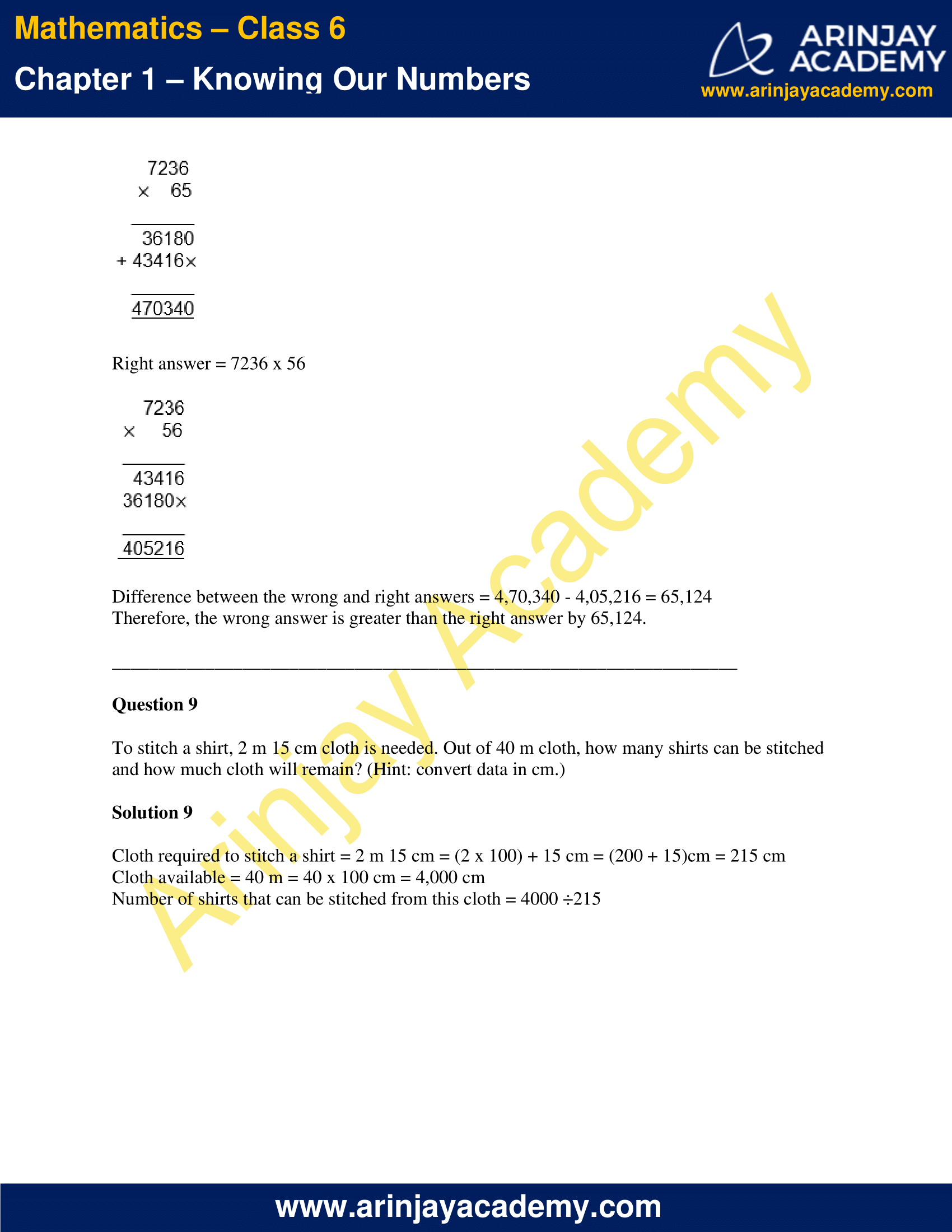• This Page 5 of Exercise 1.2, contains the following questions and their solutions  : –
• Number of boxes that can be loaded in a van which can carry 800kg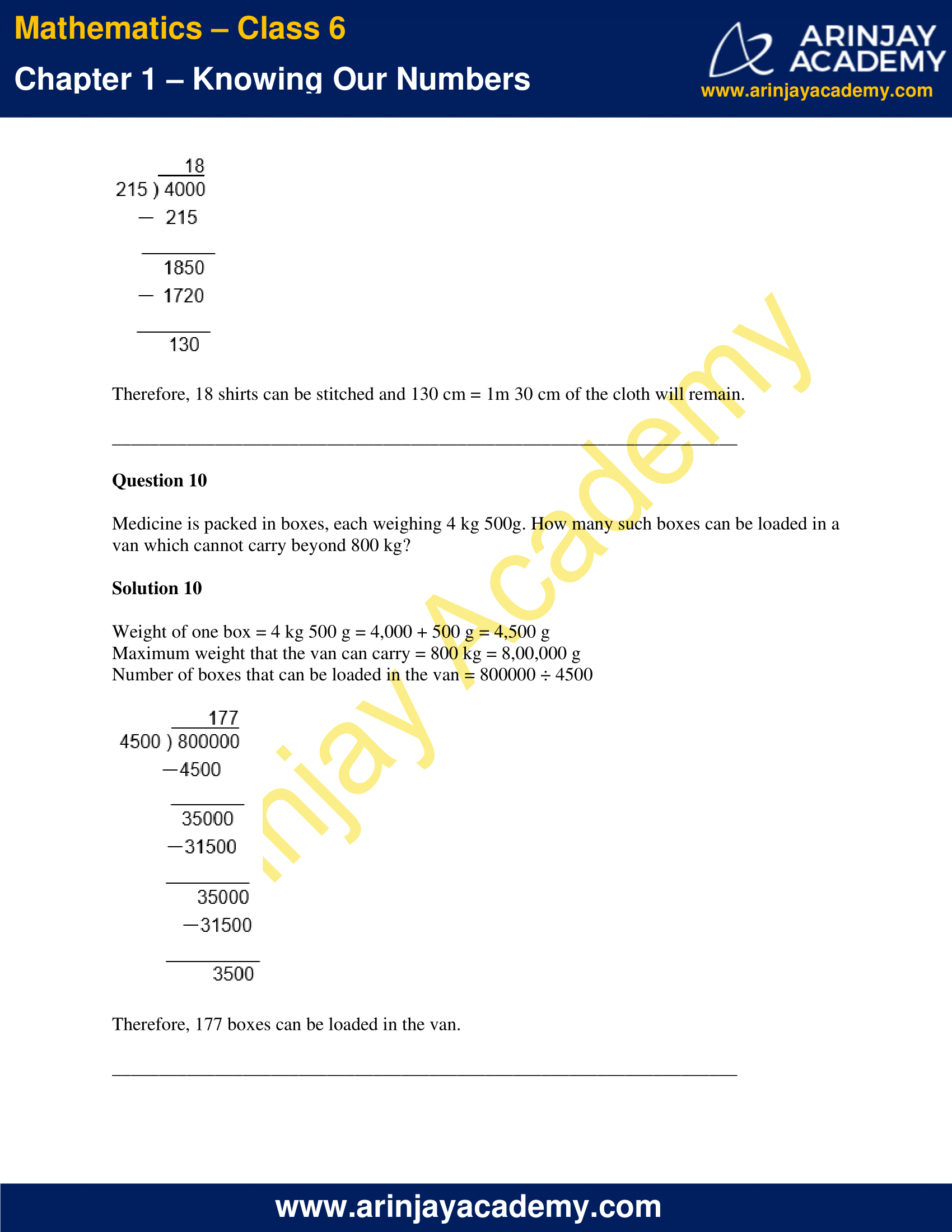• This Page 6 of Exercise 1.2, contains the following questions and their solutions  : –
• Distance covered by a student in one week to and from the school
• Number of glasses that can be filled by given amount of curd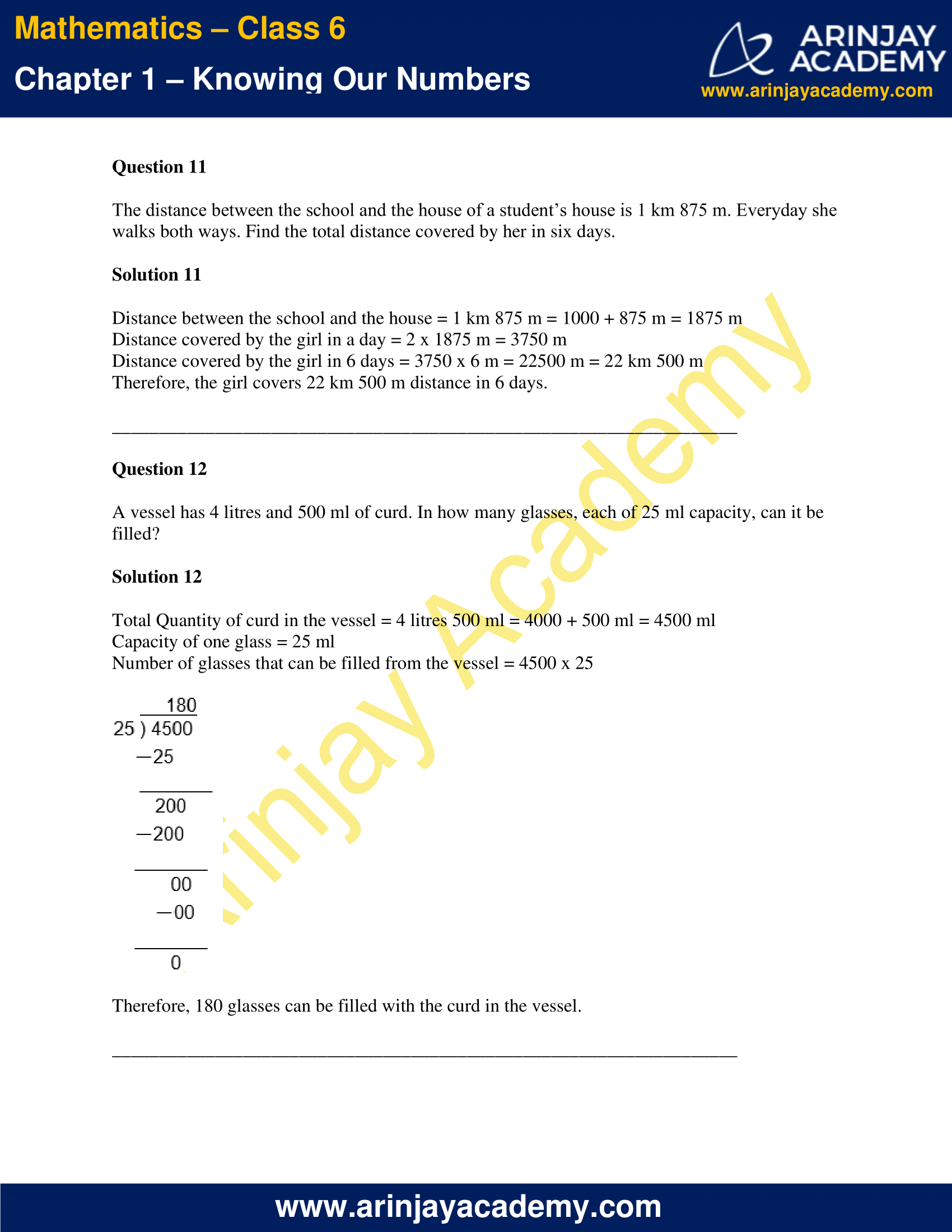### Class 6 Maths NCERT Solutions Chapter 1 – Exercise 1.3

• This Page 1 of Exercise 1.3, contains the following questions and their solutions  : –
• Estimate the sum, difference of two number by estimation
• Estimate the sum, difference of three number by estimation;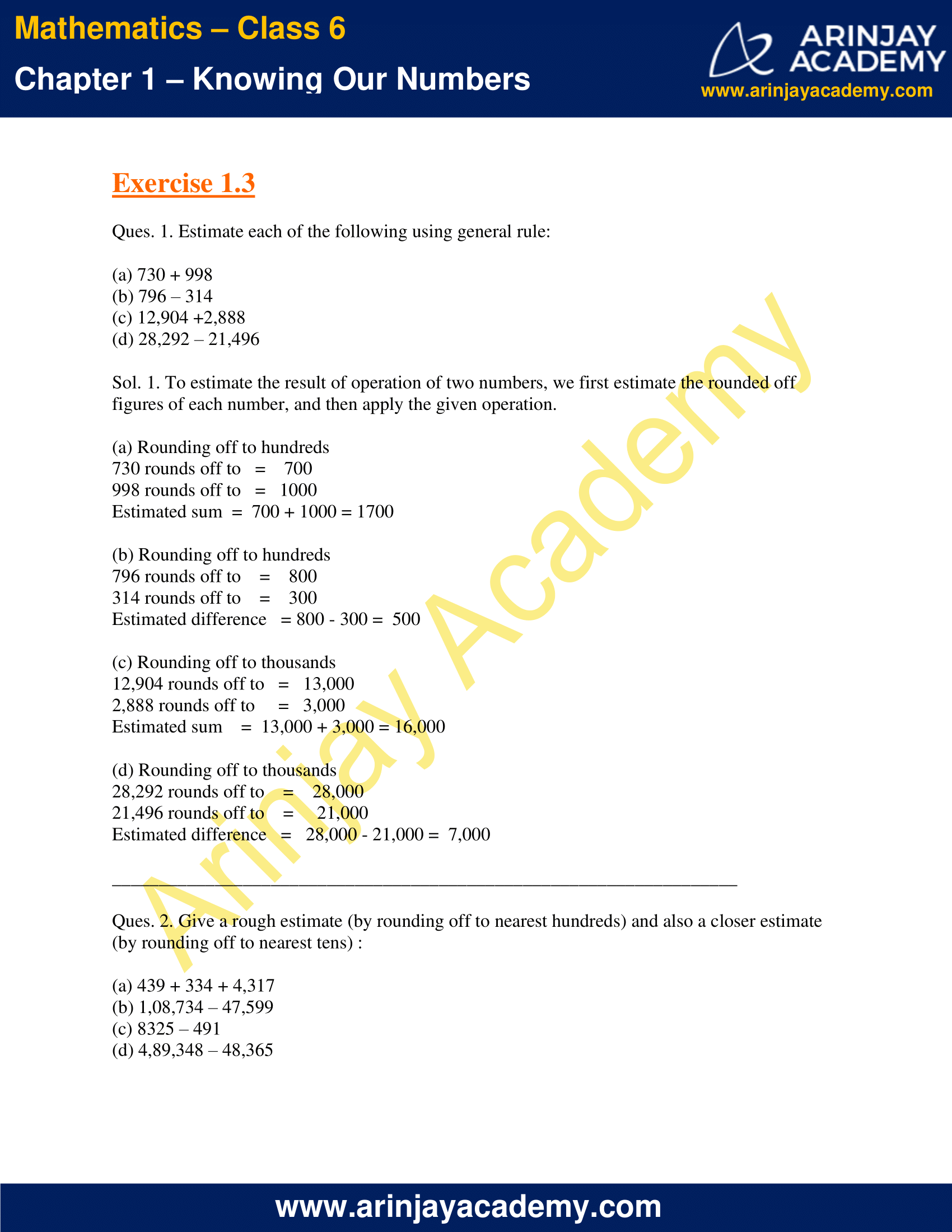• This Page 2 of Exercise 1.3, contains the following : –
• Estimate the sum, difference of three number by estimation;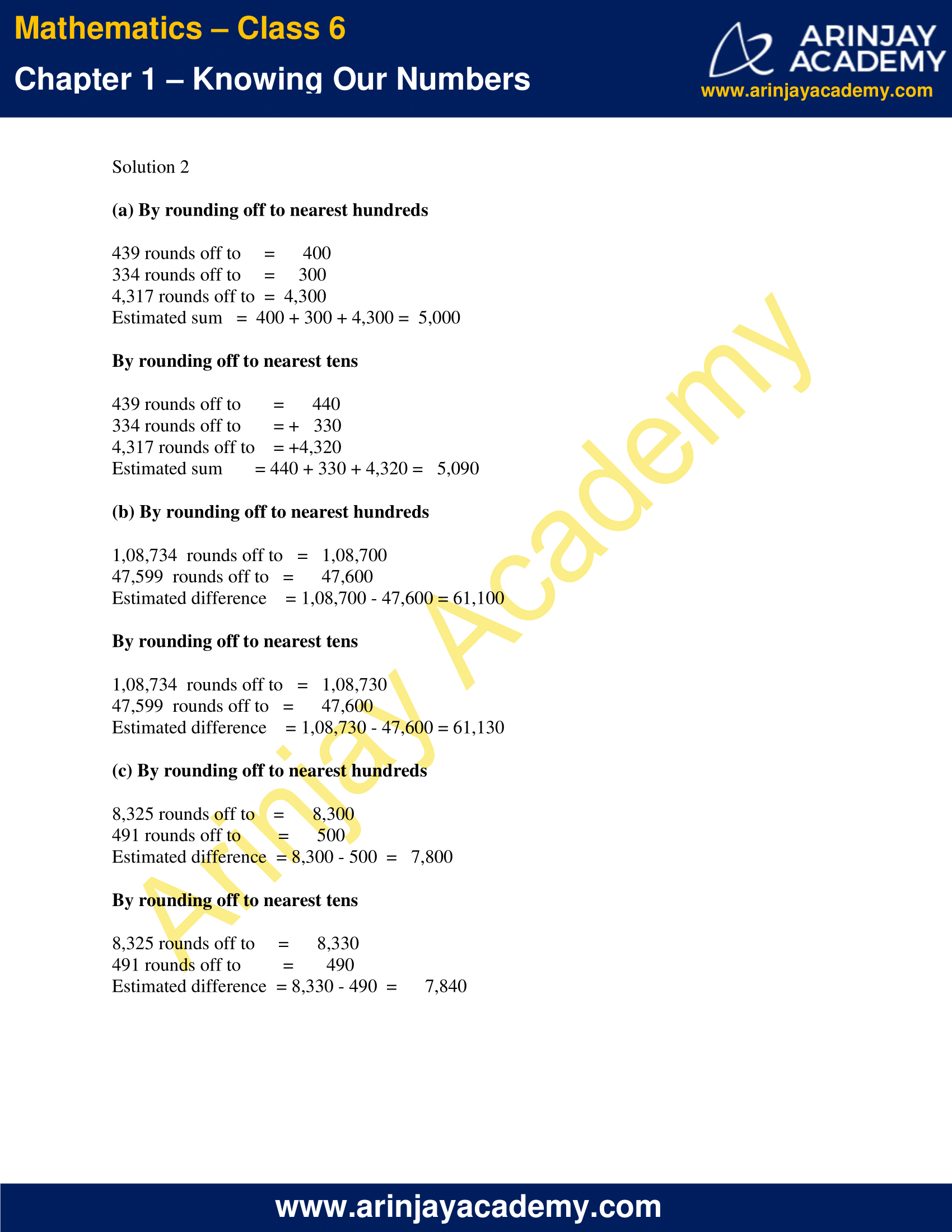• This Page 3 of Exercise 1.3, contains the following questions and their solutions  : –
• Estimate the product of two number by estimation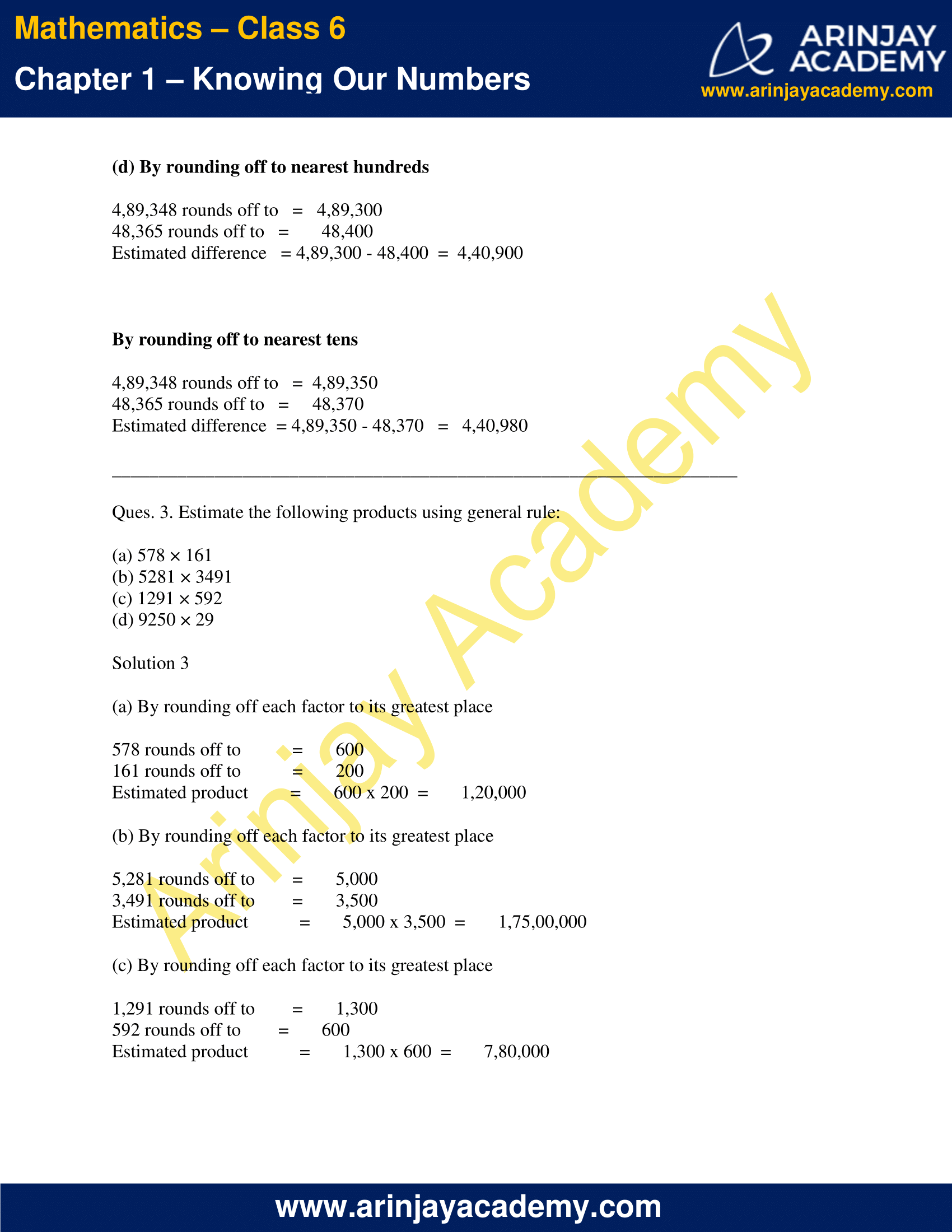• This Page 4 of Exercise 1.3, contains the following questions and their solutions  : –
• Estimate the product of two number by estimation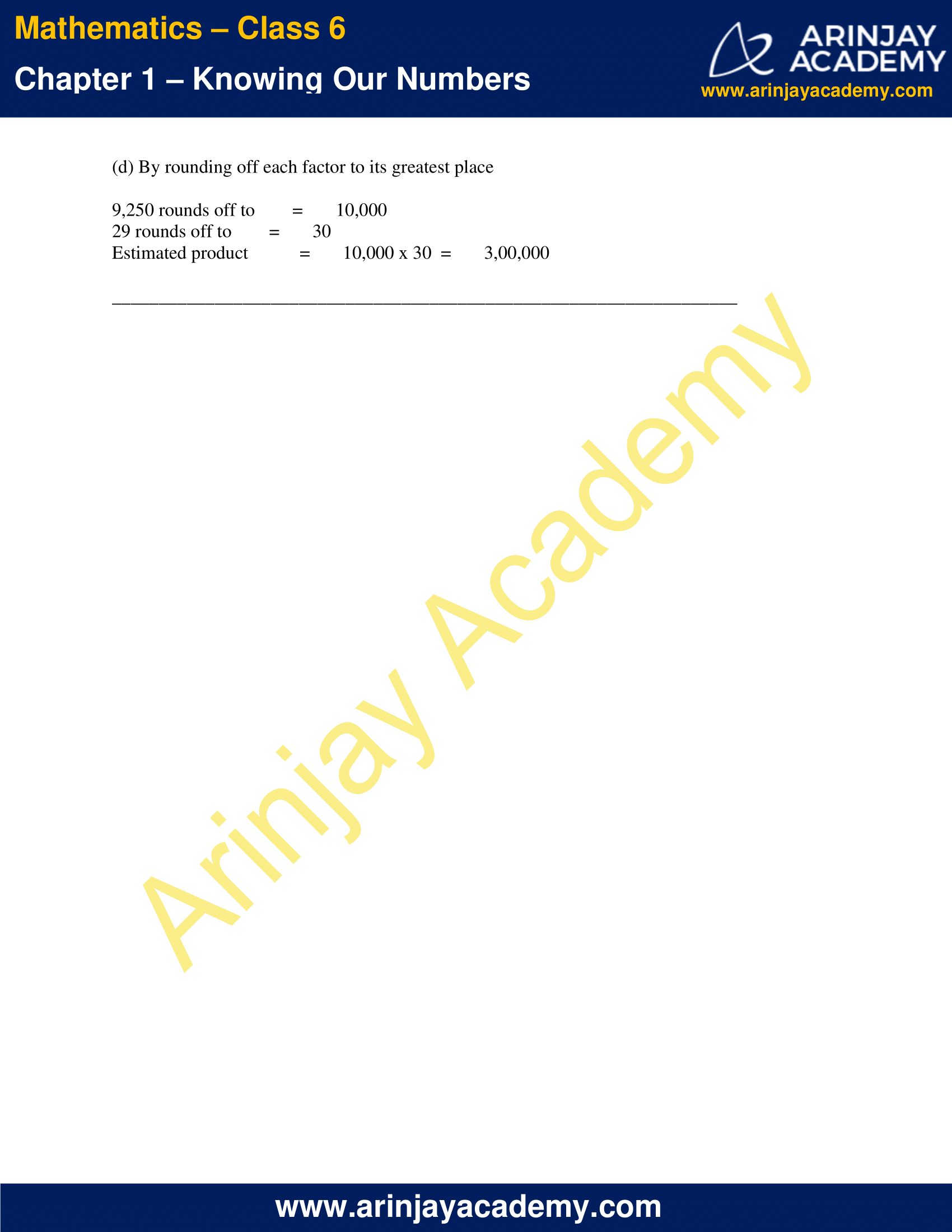### Class 6 Maths NCERT Solutions Chapter 1 – Exercise 1.1

Ques. 1. Fill in the blanks:

(a) 1 lakh = _______ ten thousand.
(b) 1 million = _______ hundred thousand.
(c) 1 crore = _______ ten lakh.
(d) 1 crore = _______ million.
(e) 1 million = _______ lakh.

Solution 1

(a) 1 lakh = 10 ten thousand
(b) 1 million = 10 hundred thousand
(c) 1 crore = 10 ten lakh.
(d) 1 crore = 10 million.
(e) 1 million = 10 lakh.

Ques. 2. Place commas correctly and write the numerals:

(a) Seventy three lakh seventy five thousand three hundred seven.
(b) Nine crore five lakh forty one.
(c) Seven crore fifty two lakh twenty one thousand three hundred two.
(d) Fifty eight million four hundred twenty three thousand two hundred two.
(e) Twenty three lakh thirty thousand ten.

Solution 2

(a) Seventy three lakh seventy five thousand three hundred seven = 73,75,307
(b) Nine crore five lakh forty one = 9,05,00,041
(c) Seven crore fifty two lakh twenty one thousand three hundred two = 7,52,21,302
(d) Fifty eight million four hundred twenty three thousand two hundred two = 58,423,202
(e) Twenty three lakh thirty thousand ten = 23,30,010

Ques. 3. Insert commas suitably and write the names according to Indian System of Numeration :

(a) 87595762
(b) 8546283
(c) 99900046
(d) 98432701

Solution 3

(a) 8,75,95,762 = Eight crore seventy five lakh ninety five thousand seven hundred sixty two
(b) 85,46,283 = Eighty five lakh forty six thousand two hundred eighty three
(c)  9,99,00,046 = Nine crore ninety nine lakh forty six
(d)  9,84,32,701 = Nine crore eighty four lakh thirty two thousand seven hundred one

Ques. 4. Insert commas suitably and write the names according to International System of Numeration :

(a) 78921092
(b) 7452283
(c) 99985102
(d) 48049831

Solution 4

(a) 78,921,092 = Seventy eight million nine hundred twenty one thousand ninety two
(b) 7,452,283 = Seven million four hundred fifty two thousand two hundred eighty three
(c) 99,985,102 = Ninety nine million nine hundred eighty five thousand one hundred two
(d) 48,049,831 = Forty eighty million forty nine thousand eight hundred thirty one

### Class 6 Maths NCERT Solutions Chapter 1 – Exercise 1.2

Question 1

A book exhibition was held for four days in a school. The number of tickets sold at the counter on the first, second, third and final day was respectively 1094, 1812, 2050 and 2751. Find the total number of tickets sold on all the four days.

Solution 1

Number of tickets sold on the first day = 1094
Number of tickets sold on the second day = 1812
Number of tickets sold on the third day = 2050
Number of tickets sold on the final day = 2751
Total number of tickets sold on all four days = 1,094 + 1,812 + 2,050 + 2,751 =  7,707
Therefore, 7,707 tickets were sold on all four days.

Question 2

Shekhar is a famous cricket player. He has so far scored 6980 runs in test matches. He wishes to complete 10,000 runs. How many more runs does he need?

Solution 2

Runs Shekhar wishes to complete = 10,000
Runs Shekhar has scored so far = 6,980
Runs required to achieve the target = 10,000 – 6,980 =   3,020
Therefore, Shekhar needs 3,020 more runs to complete 10,000 runs.

Question 3

In an election, the successful candidate registered 5,77,500 votes and his nearest rival secured 3,48,700 votes. By what margin did the successful candidate win the election?

Solution 3

Number of votes registered by the successful candidate = 5,77,500
Number of votes registered by his nearest rival = 3,48,700
Margin by which the successful candidate won =  5,77,500 – 3,48,700 =   2,28,800
Therefore, the successful candidate won by a margin of 2,28,800 votes.

Question 4

Kirti bookstore sold books worth Rs 2,85,891 in the first week of June and books worth Rs 4,00,768 in the second week of the month. How much was the sale for the two weeks together? In which week was the sale greater and by how much?

Solution 4

Worth of books sold in the first week of June = Rs 2,85,891
Worth of books sold in the second week of June = Rs 4,00,768
Sale for the two weeks together = 2,85,891 + 4,00,768 = 6,86,659
Hence, the sale was worth Rs 6,86,659 for the two weeks.

Since, 4,00,768 > 2,85,891
Therefore, the sale was more in the second week than the first week.
Sale greater than first week =  4,00,768 – 2,85,891 =  1,14,877
Therefore, the sale was greater in second week than the first week by Rs 1,14,877.

Question 5

Find the difference between the greatest and the least number that can be written using the digits 6, 2, 7, 4, 3 each only once.

Solution 5

The greatest five digit  number can be obtained by arranging the digits in descending order, and, the smallest five digit number can be obtained by arranging the digits in ascending order from the highest place to one’s place.
Hence, using the digits 6, 2, 7, 4, 3, each only once,
The greatest five digit number obtained = 76,432
The smallest five digit number obtained = 23,467
The difference between the greatest and smallest numbers so obtained = 76,432 – 23,467 = 52,965
Hence, the difference between the greatest and the least number that can be written using the digits 6, 2, 7, 4, 3 each only once is 52,965.

Question 6

A machine, on an average, manufactures 2,825 screws a day. How many screws did it produce in the month of January 2006?

Solution 6

Number of screws manufactured in a day = 2,825
Number of days in the month of January 2006 = 31
Number of screws produced in the month of January 2006 = 2,825 x 31 = 87575
Therefore, the number of screws produced in January 2006 = 87,575.

Question 7

A merchant had Rs 78,592 with her. She placed an order for purchasing 40 radio sets at Rs 1200 each. How much money will remain with her after the purchase?

Solution 7

Total amount with the merchant =  Rs 78,592
Cost of one radio set = Rs 1200
Number of radio sets ordered for purchase = 40
Total cost of purchase = Rs 1200 x 40 = Rs 48,000
Amount left with the merchant after purchase = Rs 78,592 – Rs 48,000 = Rs 30,592
Therefore, Rs 30,592 will remain with the merchant after the purchase of 40 radios.

Question 8

A student multiplied 7236 by 65 instead of multiplying by 56. By how much was his answer greater than the correct answer? (Hint: Do you need to do both the multiplications?)

Solution 8

Wrong answer = 7236 x 65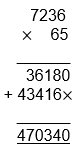Right answer = 7236 x 56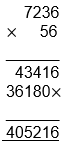Difference between the wrong and right answers = 4,70,340 – 4,05,216 = 65,124
Therefore, the wrong answer is greater than the right answer by 65,124.

Question 9

To stitch a shirt, 2 m 15 cm cloth is needed. Out of 40 m cloth, how many shirts can be stitched and how much cloth will remain? (Hint: convert data in cm.)

Solution 9

Cloth required to stitch a shirt = 2 m 15 cm = (2 x 100) + 15 cm = (200 + 15)cm = 215 cm
Cloth available = 40 m = 40 x 100 cm = 4,000 cm
Number of shirts that can be stitched from this cloth = 4000 ÷215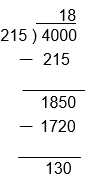Therefore, 18 shirts can be stitched and 130 cm = 1m 30 cm of the cloth will remain.

Question 10

Medicine is packed in boxes, each weighing 4 kg 500g. How many such boxes can be loaded in a van which cannot carry beyond 800 kg?

Solution 10

Weight of one box = 4 kg 500 g = 4,000 + 500 g = 4,500 g
Maximum weight that the van can carry = 800 kg = 8,00,000 g
Number of boxes that can be loaded in the van = 800000 ÷ 4500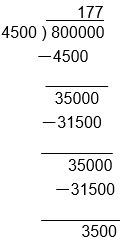Therefore, 177 boxes can be loaded in the van.

Question 11

The distance between the school and the house of a student’s house is 1 km 875 m. Everyday she walks both ways. Find the total distance covered by her in six days.

Solution 11

Distance between the school and the house = 1 km 875 m = 1000 + 875 m = 1875 m
Distance covered by the girl in a day = 2 x 1875 m = 3750 m
Distance covered by the girl in 6 days = 3750 x 6 m = 22500 m = 22 km 500 m
Therefore, the girl covers 22 km 500 m distance in 6 days.

Question 12

A vessel has 4 litres and 500 ml of curd. In how many glasses, each of 25 ml capacity, can it be filled?

Solution 12

Total Quantity of curd in the vessel = 4 litres 500 ml = 4000 + 500 ml = 4500 ml
Capacity of one glass = 25 ml
Number of glasses that can be filled from the vessel = 4500 x 25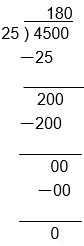Therefore, 180 glasses can be filled with the curd in the vessel.

### Class 6 Maths NCERT Solutions Chapter 1 – Exercise 1.3

Ques. 1. Estimate each of the following using general rule:

(a) 730 + 998
(b) 796 – 314
(c) 12,904 +2,888
(d) 28,292 – 21,496

Solution 1.

To estimate the result of operation of two numbers, we first estimate the rounded off figures of each number, and then apply the given operation.

(a) Rounding off to hundreds
730 rounds off to = 700
998 rounds off to = 1000
Estimated sum  =  700 + 1000 = 1700

(b) Rounding off to hundreds
796 rounds off to = 800
314 rounds off to = 300
Estimated difference = 800 – 300 =  500

(c) Rounding off to thousands
12,904 rounds off to = 13,000
2,888 rounds off to = 3,000
Estimated sum = 13,000 + 3,000 = 16,000

(d) Rounding off to thousands
28,292 rounds off to = 28,000
21,496 rounds off to = 21,000
Estimated difference = 28,000 – 21,000 =  7,000

Ques. 2. Give a rough estimate (by rounding off to nearest hundreds) and also a closer estimate (by rounding off to nearest tens) :

(a) 439 + 334 + 4,317
(b) 1,08,734 – 47,599
(c) 8325 – 491
(d) 4,89,348 – 48,365

Solution 2

(a) By rounding off to nearest hundreds

439 rounds off to = 400
334 rounds off to = 300
4,317 rounds off to = 4,300
Estimated sum = 400 + 300 + 4,300 =  5,000

By rounding off to nearest tens

439 rounds off to = 440
334 rounds off to = 330
4,317 rounds off to = 4,320
Estimated sum = 440 + 330 + 4,320 =   5,090

(b) By rounding off to nearest hundreds

1,08,734  rounds off to = 1,08,700
47,599  rounds off to = 47,600
Estimated difference = 1,08,700 – 47,600 = 61,100

By rounding off to nearest tens

1,08,734  rounds off to = 1,08,730
47,599  rounds off to = 47,600
Estimated difference = 1,08,730 – 47,600 = 61,130

(c) By rounding off to nearest hundreds

8,325 rounds off to = 8,300
491 rounds off to = 500
Estimated difference  = 8,300 – 500 = 7,800

By rounding off to nearest tens

8,325 rounds off to = 8,330
491 rounds off to = 490
Estimated difference  = 8,330 – 490 = 7,840

(d) By rounding off to nearest hundreds

4,89,348 rounds off to = 4,89,300
48,365 rounds off to = 48,400
Estimated difference = 4,89,300 – 48,400  =  4,40,900

By rounding off to nearest tens

4,89,348 rounds off to = 4,89,350
48,365 rounds off to = 48,370
Estimated difference  = 4,89,350 – 48,370 = 4,40,980

Ques. 3. Estimate the following products using general rule:

(a) 578 × 161
(b) 5281 × 3491
(c) 1291 × 592
(d) 9250 × 29

Solution 3

(a) By rounding off each factor to its greatest place

578 rounds off to = 600
161 rounds off to = 200
Estimated product = 600 x 200 = 1,20,000

(b) By rounding off each factor to its greatest place

5,281 rounds off to = 5,000
3,491 rounds off to = 3,500
Estimated product = 5,000 x 3,500 = 1,75,00,000

(c) By rounding off each factor to its greatest place

1,291 rounds off to = 1,300
592 rounds off to = 600
Estimated product = 1,300 x 600 = 7,80,000

(d) By rounding off each factor to its greatest place

9,250 rounds off to = 10,000
29 rounds off to = 30
Estimated product = 10,000 x 30 = 3,00,000

With this we come to the end of Class 6 Maths NCERT Solutions Chapter 1. We hope these helped you study your subject. You can also go to our Youtube Channel Class – 6 – Maths Video Playlist at this link Arinjay Academy Maths Class 6 – Videos on Youtube  to gain a deeper understanding of all these concepts. If you want a list of the Various Videos which are available for Class VI – You can register and access them here .